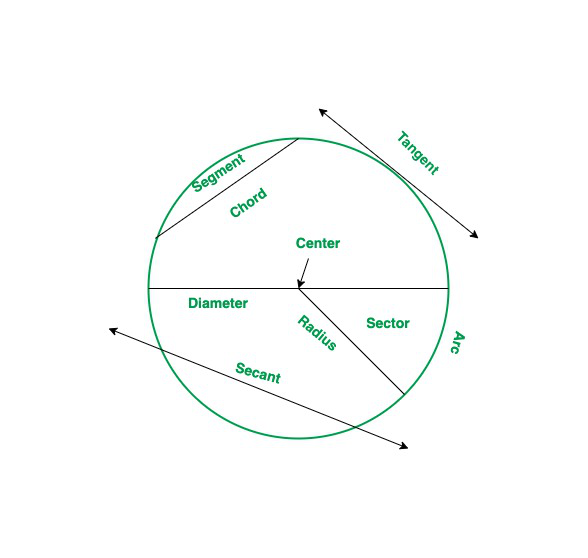# Is a Circle a Plane Shape?

• Last Updated : 19 Jan, 2022

Geometry is a mathematical subject that studies plane shapes and solid shapes along with their properties and way of representation. According to the standard definition of geometry, it is the study of shapes, structures, their size, angles formed, and representation. Euclid is widely known as the father of geometry. It is the study that explains the properties of flat shapes like circles, triangles, squares, rectangles, etc, and solid shapes like sphere, cylinder, cone, cube, cuboid, etc.

Plane shapes

A plane is a flat two-dimensional surface that extends two infinity and doesn’t have height. And, the bounded two-dimensional figure represented on these plane surfaces is known as flat shapes or plane shapes. Plane shapes comprise length and width. They do not have height as a dimension. Some of the basic plane shapes are circle, triangle, square, rectangle, etc.

Circle

A circle is a bound closed flat shape that is represented on a plane surface. A circle is a two-dimensional figure and is created by a set of points that are at a fixed distance(also known as radius) from the fixed point(also known as center) in the plane. Or was can say a circle consists of a single face and does not have edges or vertices. The number of points lying in a circle is uncountable in numbers and the direction of the line keeps changing with each point. Following are some commonly used terms in a circle:

• Circumference: It is known as the boundary of the circle.
• Radius: The distance from any point present on the boundary of the circle to the center of the circle.
• Diameter: It is a straight line joining two points present on the boundary of the circle and it always passes through the center of the circle.
• Chord: It is a line segment that touches any two points on the boundary of the circle.
• Tangent: It is a line that touches the circumference of the circle at a unique point.
• Arc: It is a part of the circumference of the circle and it is of two types minor or major arc.
• Segment: It is known as the area enclosed by the chord and the arc in a circle.
• Sector: It is known as the area enclosed by two radii and the arc in a circle.
• Secant: It is a line that divides the circle at two distinct points.

Properties

• If the radius of two circles is the same then they are known as congruent.
• The chords of a circle are always equidistant from the center.
• When a chord has a perpendicular bisector then that bisector is passing from the center of the circle.
• When two circles touch each other on exactly one point then they are known as tangent circles.
• diameter of the circle is also known as the longest chord of the circle.### Is a circle a plane shape?

Solution:

A circle is a plane shape as it is a closed figure. It is a figure that is represented on a plane surface. It is a plane figure formed by a single bounded curved line. The points in a circle are placed such that each point lies at an equal distance from the center of the circle.Therefore, each point of the circle is equidistant from the center and it is a two-dimensional structure. It can be stated that a circle is a plane shape.

### Sample Questions

Question 1: What is the diameter of a circle?

Solution:

A diameter is a chord or line segment passing through the center of the circle. Diameter is considered to be the longest chord of the circle which passes through the center. Its length equals double the radius of the circle.

Mathematically, the formula fo

e length of diameter can be written as

d = 2r

Question 2: What is the radius of a circle?

Solution:

The distance measured from the center to the circumference of the circle is defined as radius. It is the distance half of the diameter of the circle.

Question 3: What is a chord of a circle?

Solution:

In a circle, a chord is a line segment whose endpoints present on the circular arc or boundary of the circle. It Joins two endpoints in a curve. A chord can be measured in length as it has two fixed endpoints.

Question 4: How can you determine the length of a chord?

Solution:

The length of a chord can be determined by the given mathematical formulas

• The length of the chord using perpendicular distance from the center = 2 × √(r2 − d2)
• The length of the chord using trigonometry = 2 × r × sin(c/2)
My Personal Notes arrow_drop_up